• +91 9971497814
• info@interviewmaterial.com

# Chapter 4 Linear Equations in Two Variables Ex-4.1 Interview Questions Answers

### Related Subjects

Question 1 :
The cost of a notebook is twice the cost of a pen. Write a linear equation in two variables to represent this statement.
(Take the cost of a notebook to be Rs x and that of a pen to be Rs y.)

Let the cost of a notebook and a pen be x and y respectively.
Cost of notebook = 2 × Cost of pen
x = 2y
x − 2y = 0

Question 2 : Express the following linear equations in the form ax + by + c = 0 and indicate the values of a, b, c in each case:
(i)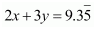(ii)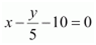(iii) − 2x + 3 y = 6

(iv) x =3(v) 2x =− 5y (vi) 3x +2 = 0

(vii) y −2 = 0 (viii) 5 = 2x

Answer 2 : (i)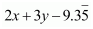= 0

Comparing this equation with ax + by =0,

= 2, = 3,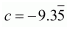(ii)Comparing this equation with ax + by c =0,

= 1, =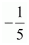, c = −10

(iii) − 2x +3 y = 6

− 2x + 3 y − 6 = 0

Comparing this equation with ax + by c =0,

= −2, b = 3, c = −6

(iv) x =3y

1x − 3y + 0 = 0

Comparing this equation with ax + by c =0,

= 1, b = −3, c = 0

(v) 2x = −5y

2x + 5y + 0 = 0

Comparing this equation with ax + by c =0,

= 2, b = 5, c = 0

(vi) 3x + 2= 0

3x + 0.y + 2 = 0

Comparing this equation with ax + by c =0,

= 3, b = 0, c = 2

(vii) y −2 = 0

0.x + 1.y − 2 = 0

Comparing this equation with ax + by c =0,

= 0, = 1, c =−2

(viii) 5 = 2x

− 2x + 0.y + 5 = 0

Comparing this equation with ax + by c =0,

= −2, = 0, c =5

Todays Deals### Chapter 4 Linear Equations in Two Variables Ex-4.1 Contributorskrishan

Name:
Email:

# Latest News# 9000 interview questions in different categories